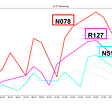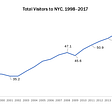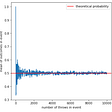# Separate Drawing of Gaussian Mixture Model

I have a 1D data which need to be separated by two .

So I used

`fitgmdist(data,2);`

and got

1. mu
2. sigma
3. component proportion

for each of the gaussian distribution.

And here is the graph. (Gray : Data, Blue : psd of GMModel from fitgmdist)

Until here, everything was okay.

So, question.

How can I separate those two gaussian distribution graph?

I tried

1. Using makedist(‘Normal’) to create each gaussian distribution.
2. Multiply by each component proportion

But somehow I wasn’t able to get the same graph overlapping picture above.

Probably I have the wrong concept of “Normalization” or “Gaussian Mixture Model”.

Any advise or site to lookup would be grateful.

— — — — — — — — — — — — — — — — — — — — — — — — — — — — — — @Image Analyst: data uploaded. thanks for the advice I’ll remember that next time :)

NOTE:-

Matlabsolutions.com provide latest MatLab Homework Help,MatLab Assignment Help for students, engineers and researchers in Multiple Branches like ECE, EEE, CSE, Mechanical, Civil with 100% output.Matlab Code for B.E, B.Tech,M.E,M.Tech, Ph.D. Scholars with 100% privacy guaranteed. Get MATLAB projects with source code for your learning and research.

You did something like this:

`x = [randn(4000,1)/2; 5+2*randn(6000,1)];f = fitgmdist(x,2);histogram(x,'Normalization','pdf')xgrid = linspace(-4,12,1001)';hold on; plot(xgrid,pdf(f,xgrid),'r-'); hold off`

You can duplicate the pdf values by doing something like this:

`n1 = makedist('normal',f.mu(1),sqrt(f.Sigma(1)));n2 = makedist('normal',f.mu(2),sqrt(f.Sigma(2)));p = f.ComponentProportion;y = p(1)*pdf(n1,xgrid) + p(2)*pdf(n2,xgrid);hold on; plot(xgrid,y,'c--'); hold off`

One thing to watch out for. In probability and statistics, it’s common to write the standard deviation of a univariate normal distribution as the Greek letter sigma.

--

--

--

## More from Technical Source

Simple! That is me, a simple person. I am passionate about knowledge and reading. That’s why I have decided to write and share a bit of my life and thoughts to.

Love podcasts or audiobooks? Learn on the go with our new app.

## ICM Media Final Project -First Part## Measuring Labelling Quality with IOU and F1 Score## Excelling at Excel!## A Vision Zero Action Plan comes to Kirkwood, MO## Underground Apple## Data-Informed Planning in California## Analyzing data of New York City from Airbnb using Pandas.## So you want to visit Berlin?## Technical Source

Simple! That is me, a simple person. I am passionate about knowledge and reading. That’s why I have decided to write and share a bit of my life and thoughts to.

## Predicting the probability of default for Mortgage-Backed Securities## The Beta Distribution explained through Binomial and Bernoulli Distribution